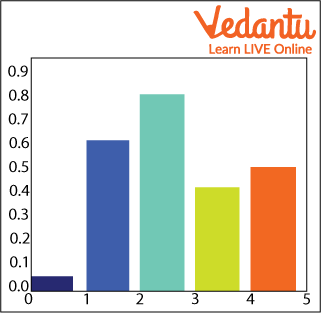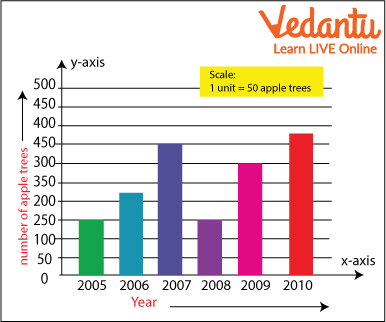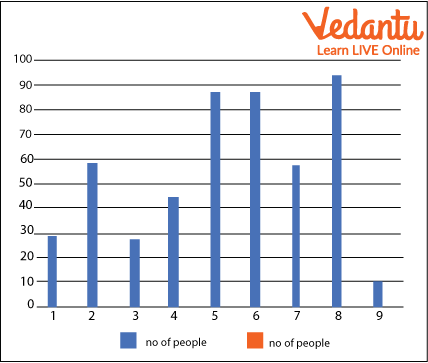Courses
Courses for Kids
Free study material
Free LIVE classes
More

# Bar Graph ScaleLIVE
Join Vedantu’s FREE Mastercalss

## What is Bar Graph?

Bar graphs are the visual representation of knowledge, within the form of vertical or horizontal rectangular bars, where the length of bars are proportional to the measure  of knowledge .  A bar chart  is the simplest and easiest and understable method to represent the data. It helps  to research , and make decisions to support  it.  It's  very helpful in making any kind of decision.

Bar graphs are nothing but  a visible  representation of data,  within the  form of vertical or horizontal rectangular shaped bars, where the length of bars is proportional to the measure  of knowledge .  They're  also known as bar charts.

## Terms Includes in Bar Chart

The terms are as follows:

• Vertical axis

• Horizontal axis

• The bar graph’s title

• The title of the horizontal axis

• The title of the vertical axis

• The categories on  the actual  axis indicate what each bar represents.

• The bar graph’s scale

• Types of Bar Graphs

The bar graphs  are often  vertical or horizontal.  The first  feature of any bar graph is its length or height.

Bar graphs usually show categorical and numeric variables arranged  in school  intervals. They  contain  an axis and a series of bars which are labelled horizontally or vertically. The bars represent distinctive values of a variable or commonly or the frequencies of the distinct values.  The amount  of values on the x-axis of a bar graph or the y-axis of a column graph is called the scale.

The types of Bar Graph Charts that can be made are as follows:

1.Vertical  Bar Graph Chart

2. High Bar Chart Graph

Even though the graph can be plotted using horizontal or vertical lines,  the most  usual type of bar graph that is used is the vertical bar graph. The orientation of the x-axis and y-axis are changed  counting on  the type of vertical and horizontal bar chart.  aside from  the vertical and horizontal bar graph,  the 2  different types of bar charts are:

• Grouped  bar chart

• Stacked  bar chart

Now,  allow us to  discuss the four different types of bar graphs.

## How to Draw a Bar Graph?

Let us consider an example,  we have  four different types of pets,  like  cat, dog, rabbit, and hamster  and therefore the  corresponding numbers are 22, 39, 5 and 9 respectively.

In order to visually represent the data using the bar graph,  we'd like  to follow the steps given below.

Step 1: Right the title of the  bar chart .

Step 2: Draw the horizontal axis and vertical axis on the graph sheet. (For example,  sorts of  junk food)

Step 3: Now, label the horizontal axis.

Step 4: Write the names on the horizontal axis,  like  burger, pizza, chips, etc.

Step 5: Now, label the vertical axis. (For example,  the amount  of eaters)

Step 6: Then, decide  the size  range for the given data.

Step 7: Finally, draw the  bar chart  that should represent each category of the food with their numbers.

## Bar Graph Scale

Scale means  the amount  used to represent one unit length of a bar.  For instance ,  the size  for the bar graph shown here is 1 unit length = 100 eaters.

## Bar Chart ExamplesBar Chart 1Bar Chart 2

## Examples of Bar Graph

1. Make a bar graph using the given information

 No. of people 20 45 25 45 48 85 58 96 100 food samosa pizza chips burger momo Kfc chicken French fries pakora Onion spring

Graph 1

## Solved Questions

1. Make a bar graph using the given information

 No. of people 28 58 27 44 88 87 58 94 10 Colour red black yellow green purple orange blue pink violetGraph 2

## Summary

In this article we have studied the bar diagram even though we have covered the topic like how to make bar diagrams and the terms related to it. We have also covered the various types of diagrams in this article.

Last updated date: 29th Sep 2023
Total views: 67.8k
Views today: 1.67k

## FAQs on Bar Graph Scale

1. What is a bar diagram?

Bar graphs are nothing but  a visible representation of data,  within the  form of vertical or horizontal rectangular shaped bars, where the length of bars is proportional to the measure  of knowledge .  They're  also known as bar charts.

2. What are the terms related to the bar diagram?

Scale and Title are some of the terms that are related to a bar diagram.

3. Name any two types of bar diagrams?

Horizontal and vertical Diagram.